UPSC  >  Clocks and Calendar- Examples (with Solutions); Arithmetic; Quantitative Reasoning

# Clocks and Calendar- Examples (with Solutions); Arithmetic; Quantitative Reasoning - Notes | Study UPSC Prelims Paper 2 CSAT - Quant, Verbal & Decision Making - UPSC

 1 Crore+ students have signed up on EduRev. Have you?

Clocks

The dial of a clock is a circle whose circumference is divided into 12 parts, called hour spaces. Each hour
space is further divided into 5 parts, called minute’s spaces. This way, the whole circumference is divided into
12 × 5 = 60 minute spaces.

If we consider clock as a circular track and the two hands of clock minute hand
and hour hand are just like two players running in the same direction. Let total
length of the track is 360o and minute hand completes one full round in 1 hour
while hour hand cover full round in 12 hours.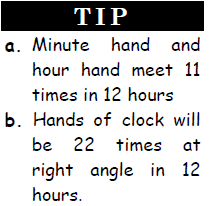Speed of hour hand =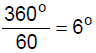per second

Speed of minute hand =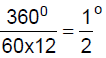per second

Since they are moving in the same direction, so the relative speed of both the hands with respect to each
other =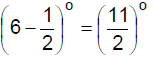per second.

Time taken by minute hand to overtake hour hand =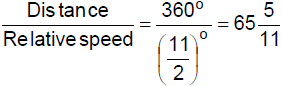There are 4 types of problems on clocks:
1. To calculate the angle between the two hands when time is given.
2. To calculate the time when both the hands will be at some angle.
3. Concept of slow and fast clocks.
4. Overall gain/loss

Calculating the angle
The angle between the two hands is given by the following formula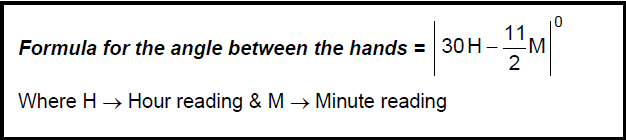Ex.1 Calculate the angle between the two hands of clock when the clock shows 5 : 25 p.m.

Sol. Given time = 5 : 25 p.m. Hence H = 5 and M = 25
We can apply the following direct formula to find the angle between the hands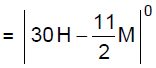Required angle =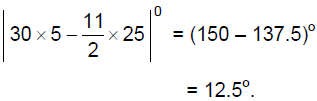Alternative Method:

Since at 5 : 25 the minute hand will be at 5 and the angle between them will be same as the distance
covered in degree by the hour hand in 25 minutes

Required angle = distance of hour hand = speed × time =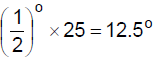Calculating the time

To calculate the time when both the hands will be at some angle
In one minute the net gain of minute hand over hour hand =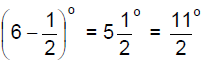If the gain is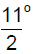then the time is 1 min.
If the gain is 1o then the time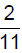is min
If the gain is xo then the time is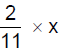min.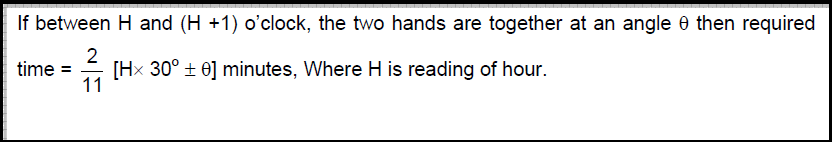Ex.2 At what time between 4 and 5 o’clock are the hands of the clock together?

Sol. Method 1: At 4 o’clock, the hour hand is at 4 and the minute hand is at 12. It means that they are 20
min spaces apart. To be together, the minute hand must gain 20 minutes over the hour hand. Now, we
know that 55 min. are gained in 60 min.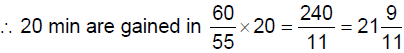min.

Therefore, the hands will be together at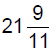min past 4.

Alternate method: Using the formula: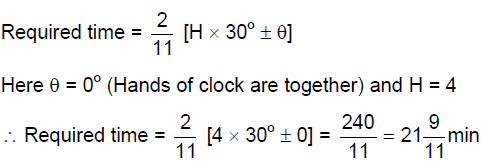min.

Therefore, the hands will be together atmin past 4.

Another formula: Between H and (H + 1) o’clock, the two hands will be together at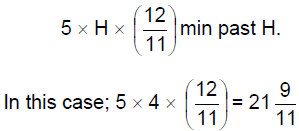min past 4

Ex.3 At what time between 4 and 5 o’clock will the hand of clock be at right angle?

Sol. At 4o’clock there are 20 min. spaces between hour and minute hands. To be at right angle, they should
be 15 min spaces apart.

So, there are two cases:

Case I: When the minute hand is 15 min spaces behind the hour hand.

To be in this position, the min hand should have to gain 20 – 15 = 5 min spaces.

Now, we know that 55 min spaces are gained in 60 min.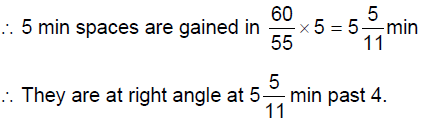Case II: When the minute hand is 15 min spaces ahead of the hour hand.

To be in this position, the min hand should have to gain 20 + 15 = 35 min spaces.

Now, we know that 55 min spaces are gained in 60 min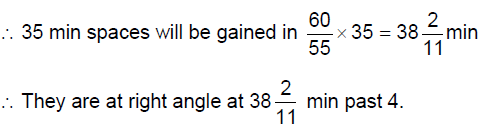Alternate method
As the hands of the clock are at right angle therefore θ = 900
Also time is between 4 and 5 o’clock, no of hours = 4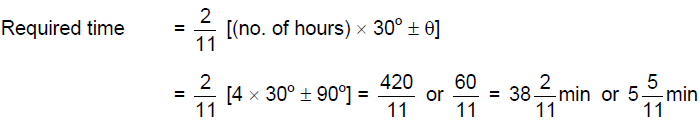∴ They are at right angle at min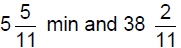min past 4.

Another Formula: Between x and (x + 1) o’clock the two hands are rt angle at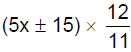min past x

In the case; they will be at right angle at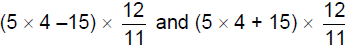min past 4

ormin past 4.

Concept of slow and fast clocks
Too Fast and Too Slow:

If a watch indicates 9.20 when the correct time is 9.10, it is said to be 10 minutes too fast. And if it indicates
9.00 when the correct time is 9.10, it is said to be 10 minute too slow.

Ex.4 Two clocks are set at 1 p.m. Fast clock gains 1 min for every hour. Find the time when the fast
clock shows 6 p.m.

Sol. For every 60 min of true clock, the fast clock will show 61 min.
For 61 minutes of fast clock, true time = 60 minutes
For 300 minutes (5 hrs) of fast clock, true time =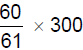=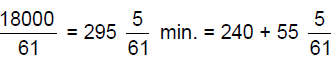Actual time in the true clock =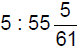hrs.

Overall gain/loss
After every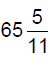min. =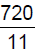min. the two hands will coincide. If the hands of a clock coincide every ‘x’ min,
then
Gain/loss per day by a watch, is given by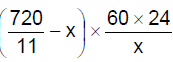[If answer is (+) then there will be gain and if (–) then there will be loss.]

Calendar

There are 12 months in 1 year.

Jan –31 (days), Feb –28; 29 (for a leap year), Mar –31, Apr – 30, May –31,

June – 30; July – 31; Aug – 31; Sept –30; Oct – 31; Nov –30; Dec – 31.

Total 365 (ordinary year); 366 days (for a leap year)

To find the number of weeks in an ordinary year: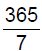= 52 weeks + 1 odd day (remainder)

To find the number of weeks in a leap year: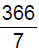= 52 weeks + 2 odd days (remainder)

Leap year: It is so called as it comes after a leap of 3 years from the previous leap year.

Method to find whether a given year is a leap year or an ordinary year.

Every year which is not a century year (i.e. which is not a multiple of 100) is a leap year if and only if it is
completely divisible by 4.

Every century year is a leap year if and only if it is completely divisible by 400 or is an integral multiple of 400
(i.e. the remainder ought to be 0).
e.g. 2000 is a leap year.
1900 is not.
1996 is a leap year, 1998 is not.

Odd day’s concept
To find the number of odd days in a century

A century, i.e. 100 year has 76 ordinary year and 24 leap year.
= [(76 × 52) weeks + 76 days] – [(24 × 52) weeks + 24 × 2 days]
= 5200 weeks + 124 days
= 5200 weeks + 17 weeks + 5 odd days
= 5217 weeks + 5 odd days

Therefore, 100 years contain 5 odd days.
Now, (i) 200 years contain 5 × 2 = 10, i.e., 3 odd days.
(ii) 300 years contain 5 × 3 = 15 i.e., 1odd day.
(iii) 400 years contain 5 × 4 + 1 = 21, i.e., no odd day.
Similarly, 800, 1200 years etc. contain no odd day.

Note: (i) 5 × 2 = 10 days = 1 week + 3 days i.e., 3 odd days
(ii) 5 × 3 = 15 days = 2 weeks + 1 day i.e. 1 odd day.
(iii) 400th year is a leap year therefore one additional day is added.

Odd days and their numeral values
When we have to calculate the number of days on any particular Extra Days and their numeral Values
0 → Sunday
1 → Monday
2 → Tuesday
3 → Wednesday
4 → Thursday
5 → Friday
6 → Saturday

Ex.5 Father of Nation Mahatma Gandhi died on 30th January 1948. What was the day on which he
died?

Sol. Up to 1600 AD we have 0 odd days, up to 1900 AD we have 1 odd day. Now for in 47 years we have
11 leap years and 36 normal years.
Odd days from 1901 to 1947 = 11 x 2 +36 x1 = 22 + 36 =58 odd days
= 8 weeks + 2 odd days

Total odd days up to 31st December 1947 = 1 + 2 = 3 odd days
30 days of January contain only 4 weeks + 2 odd days
So 30th January 1948 has total 5 odd days
Day on 30th January 1948 = Friday.

Ex.6 How does the number of odd days help us in finding the day of a week? (Please take care of this point)
When a specific day is given:
Suppose a question like:
Jan 1, 1992 was Wednesday. What day of the week will it be on Jan 1,
1993? If you recall, 1992 being a leap year it has 2 odd days. So, the above said day will be two days
beyond Wednesday, i.e., it will be Friday.

When no specific day is given:
Here, we count days according to number of odd days.
Sunday for 0 odd day, Monday for 1 odd day and so on. (i.e. from 0 to 6; 6 being
Saturday)
Suppose someone asks you to find the day of the week on 12th January 1979.
12th Jan, 1979 means 1978 year + 12 days
Now, 1600 years have 0 odd day.

300 years have 1 odd day
78 years have 59 ordinary year + 19 leap years = 6 odd days.
Total no. of odd days = 0 + 1 + 6 + 12 = 19 or 5 odd days. So, the day was “Friday”.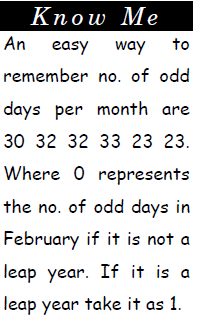Points not to be ignored:

1. 400th year is a leap year or a century multiple of 400 is a leap year, rests are not.

2. 100 years has 5 odd days
200 years has 3 odd days
300 years has 1 odd day
400 years has 0 odd days. And so on.

The document Clocks and Calendar- Examples (with Solutions); Arithmetic; Quantitative Reasoning - Notes | Study UPSC Prelims Paper 2 CSAT - Quant, Verbal & Decision Making - UPSC is a part of the UPSC Course UPSC Prelims Paper 2 CSAT - Quant, Verbal & Decision Making.
All you need of UPSC at this link: UPSC

## UPSC Prelims Paper 2 CSAT - Quant, Verbal & Decision Making

67 videos|50 docs|151 tests
 Use Code STAYHOME200 and get INR 200 additional OFF

## UPSC Prelims Paper 2 CSAT - Quant, Verbal & Decision Making

67 videos|50 docs|151 tests

### How to Prepare for UPSC

Read our guide to prepare for UPSC which is created by Toppers & the best Teachers

Track your progress, build streaks, highlight & save important lessons and more!

,

,

,

,

,

,

,

,

,

,

,

,

,

,

,

,

,

,

,

,

,

,

,

,

;